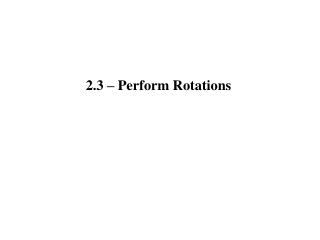DownloadDownload Presentation2.3 – Perform Rotations

# 2.3 – Perform Rotations

Télécharger la présentation## 2.3 – Perform Rotations

- - - - - - - - - - - - - - - - - - - - - - - - - - - E N D - - - - - - - - - - - - - - - - - - - - - - - - - - -
##### Presentation Transcript

1. 2.3 – Perform Rotations

2. Transformation that turns a figure about a fixed point Rotation: Center of Rotation: The point that the rotation happens around Angle of Rotation: How many degrees clockwise or counterclockwise a shape is turned

3. A rotation about a point P through an angle of x° maps every point Q in the plane to a point such that: • If Q is not the center of rotation, then • and x°

4. A rotation about a point P through an angle of x° maps every point Q in the plane to a point such that: • If Q is the center of rotation, then Q

5. Example #1: ROTATIONS ON A GRID Consider what you know about rotation, a motion that turns a shape about a point. Does it make any difference if a rotation is clockwise ( ) versus counterclockwise ( )? If so, when does it matter? Are there any circumstances when it does not matter? And are there any situations when the rotated image lies exactly on the original shape?

6. Investigate these questions as you rotate the shapes below about the given point below. Use tracing paper if needed. Be prepared to share your answers to the questions posed above.

7. - 180° doesn’t matter if you go clockwise or counterclockwise - 90° counterclockwise is the same as 270 clockwise - 360° rotates all the way around and matches the original shape

8. (5,5) (5,2) (2,2)

9. (5,5) (5,2) (2,2)

10. (5,5) (5,2) (2,2)

11. Example #3: State if the rotation about the origin is 90°, 180°, or 270° counter-clockwise.

12. 4.Find the value of each variable in the rotation. 4 x = z = 3 y = z + 2 y = 3 + 2 y = 5

13. 4.Find the value of each variable in the rotation. 4s = 24 s = 6 r = 2s – 3 r = 2(6) – 3 r = 12 – 3 r = 9